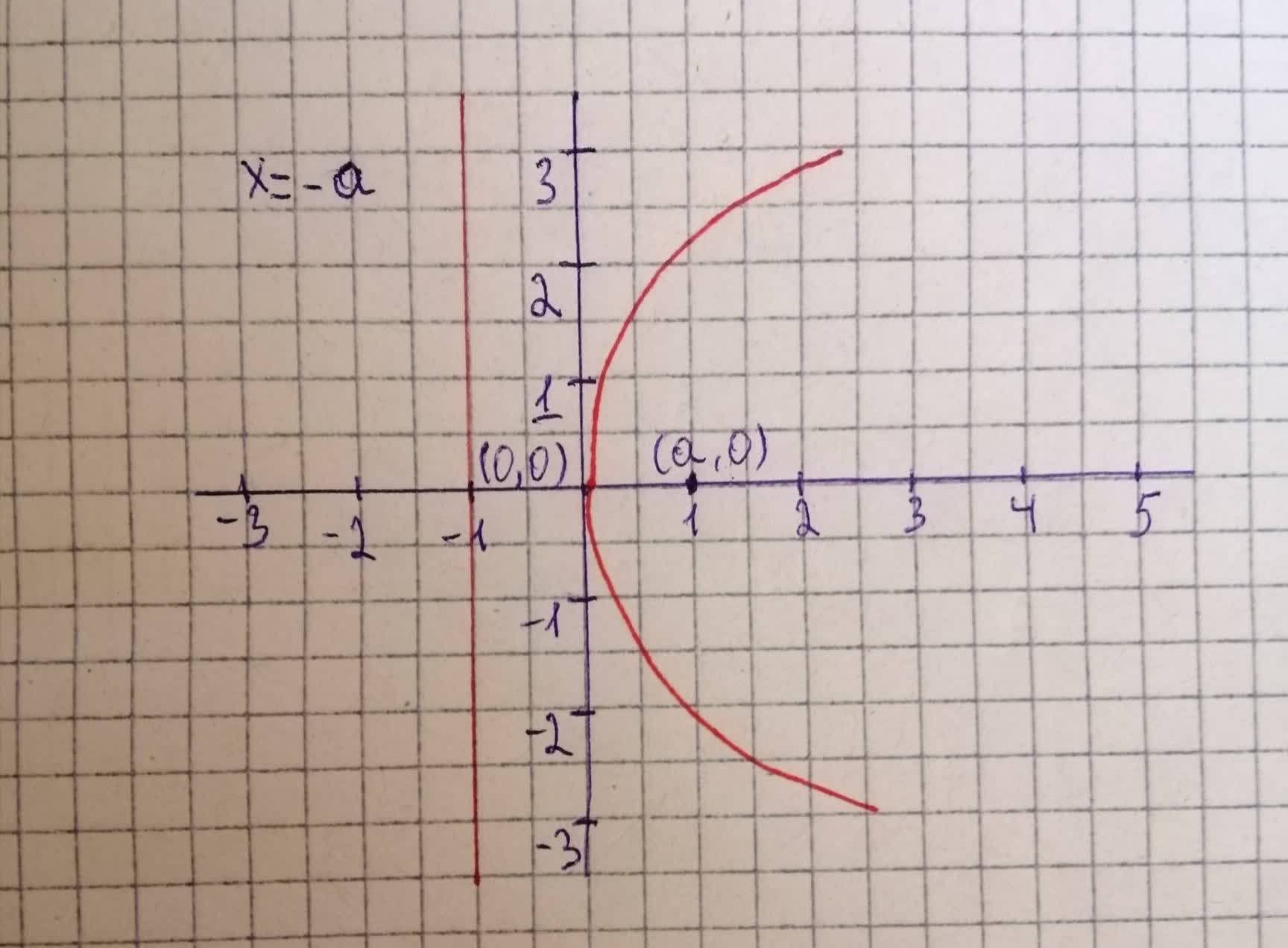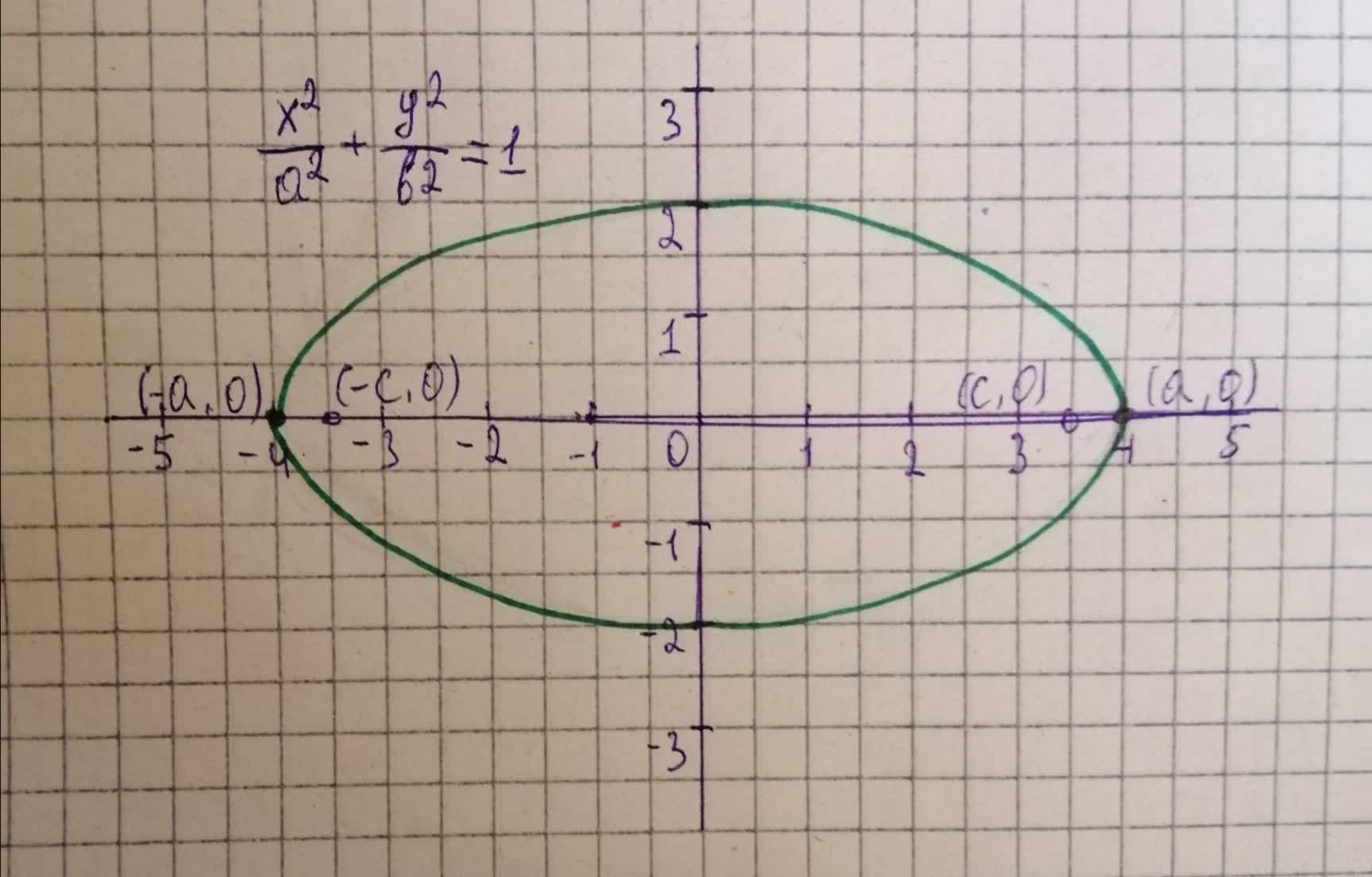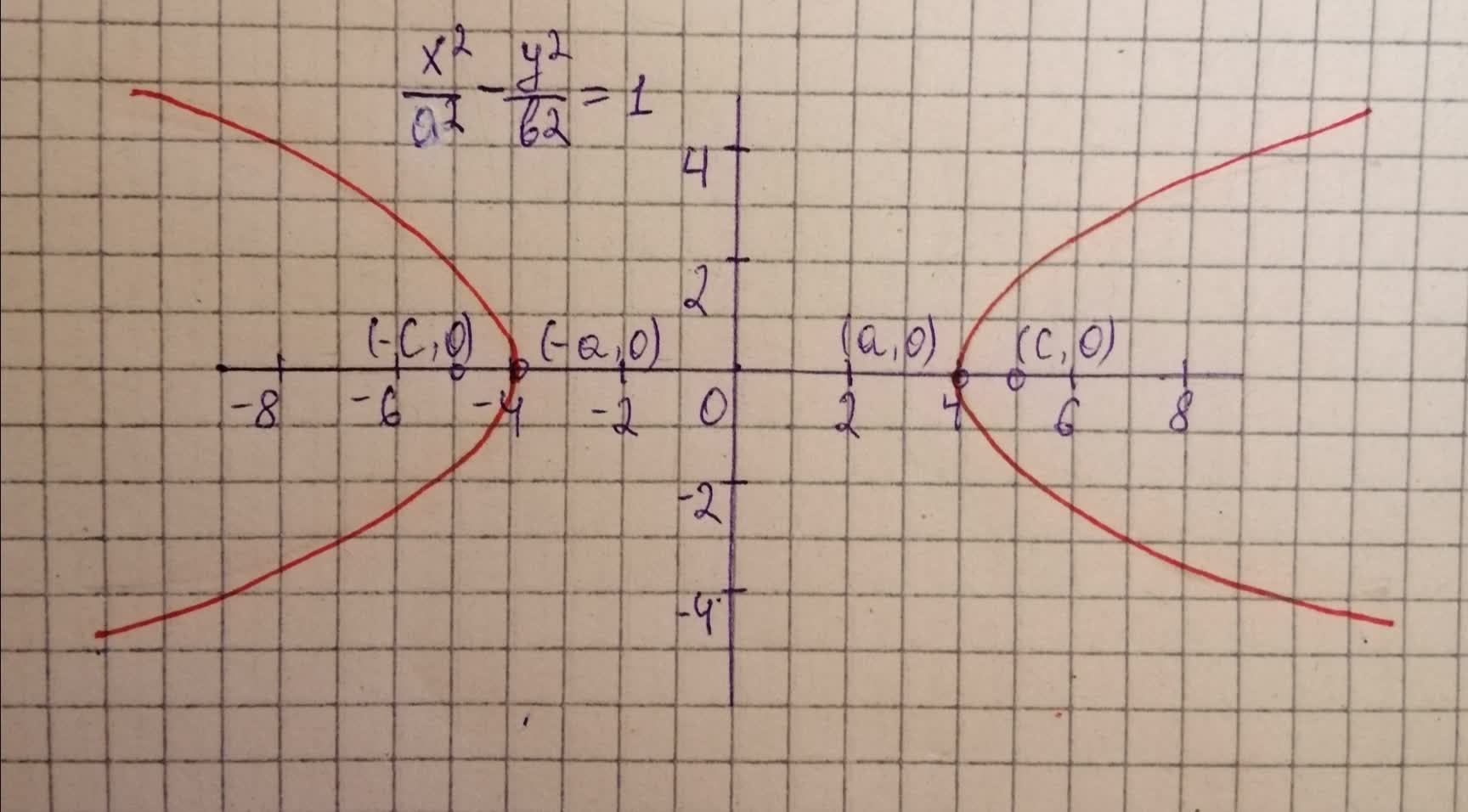Sketch the three basic conic sections in standard position with vertices and foci on the x-axis.York 2021-08-10 Answered
Sketch the three basic conic sections in standard position with vertices and foci on the x-axis.

• Questions are typically answered in as fast as 30 minutes

Solve your problem for the price of one coffee

• Math expert for every subject
• Pay only if we can solve itcomentezq

Step 1
The basic three conic sections are parabola. ellipse and hyperbola.
The standard equation of the parabola having its vertex and focus on the x-axis is
$$\displaystyle{y}^{{{2}}}={4}{a}{x}.$$
The focus of the parabola is $$\displaystyle{\left({a},\ {0}\right)}$$ and the vertex is $$\displaystyle{\left({0},\ {0}\right)}.$$
Sketch the graph of the parabola $$\displaystyle{y}^{{{2}}}={4}{a}{x}$$ as shown below.From the above figure, it is observed that the standard parabola is symmetric about x-axis and opening right side.
Step 2
The standard equation of the ellipse having its vertices and foci on the x-axis is
$$\displaystyle{\frac{{{x}^{{{2}}}}}{{{a}^{{{2}}}}}}+{\frac{{{y}^{{{2}}}}}{{{b}^{{{2}}}}}}={1}.\ {a}^{{{2}}}={b}^{{{2}}}+{c}^{{{2}}}$$
The vertices of the ellipse are $$\displaystyle{\left(\pm\ {a},\ {0}\right)}$$ and the foci are $$\displaystyle{\left(\pm\ {c},\ {0}\right)}.$$
Draw the ellipse $$\displaystyle{\frac{{{x}^{{{2}}}}}{{{a}^{{{2}}}}}}+{\frac{{{y}^{{{2}}}}}{{{b}^{{{2}}}}}}={1}$$ as shown below.From the above figure, it is observed that the ellipse has the x-axis as the major axis and the y-axis as the minor axis. And it is called the horizontal ellipse.
Step 3
The standard equation of the hyperbola having its vertices and foci on the x-axis is
$$\displaystyle{\frac{{{x}^{{{2}}}}}{{{a}^{{{2}}}}}}-{\frac{{{y}^{{{2}}}}}{{{b}^{{{2}}}}}}={z}.\ {b}^{{{2}}}={c}^{{{2}}}-{a}^{{{2}}}.$$
The vertices of the hyperbola are $$\displaystyle{\left(\pm\ {a},\ {0}\right)}$$ and the foci are $$\displaystyle{\left(\pm\ {c},\ {0}\right)}$$
Draw the hyperbola $$\displaystyle{\frac{{{x}^{{{2}}}}}{{{a}^{{{2}}}}}}-{\frac{{{y}^{{{2}}}}}{{{b}^{{{2}}}}}}={1}$$ as shown below.From the above figure, it is observed that the hyperbola has the x-axis as major axis and the y-axis as a minor axis.